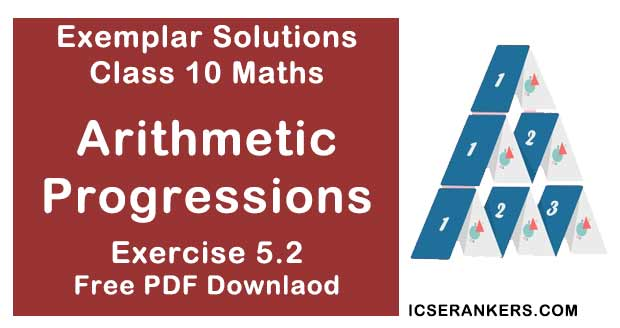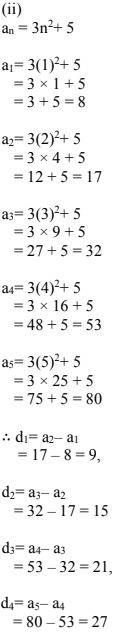# Chapter 5 Arithmetic Progressions NCERT Exemplar Solutions Exercise 5.2 Class 10 MathsChapter Name NCERT Maths Exemplar Solutions for Chapter 5 Arithmetic Progressions Exercise 5.2 Book Name NCERT Exemplar for Class 10 Maths Other Exercises Exercise 5.1Exercise 5.3Exercise 5.4 Related Study NCERT Solutions for Class 10 Maths

### Exercise 5.2 Solutions

1. Which of the following form an AP ? Justify your answer.
i. −1, −1, −1, −1, ...
ii. 0, 2,0, 2, ...
iii. 1,1, 2, 2,3,3, ...
iv. 11, 22,33, ...
v. 1/2, 1/3, 1/4, ......
vi. 2, 22 , 23, 24 , …….
vii. √3, √12, √27, √48, ......

Solution

(i) -1, -1, -1, -1,.....
We have
a1 = -1,
a2  = -1,
a3 = -1 and a4 = -1
a2 - a1 = 0
a3 - a2 = 0
a4 - a3 = 0
As the difference of successive terms is same, therefore given list of numbers from an A.P.

(ii) 0, 2, 0, 2, .....
We have
a1 = 0,
a2 = 2,
a3 = 0 and a4 = 2
a2 - a1 = 2
a3 - a2 = -2
a4 - a3 = 2
The difference of successive terms is not same, therefore given list of numbers does not form an AP.

(iii) 1, 1, 2, 2, 3, 3 ......
We have
a1 = 1,
a2 = 1,
a3 = 2 and a4 = 2
a2 - a1 = 0
a3 - a2 = 1
The difference of successive terms is not same, therefore given list of number does not form an AP.

(iv) 11, 22, 33, ........
We have
a1 = 11,
a2 = 22 and a3 = 33
a2 - a1 = 11
a3 - a2 = 11
The difference of successive terms is same, therefore given list of numbers form an AP

(v) 1/2, 1/3, 1/4, .......
We have
a1 = 1/2,
a2 = 1/3 and a3 = 1/4
a2 - a1 = -1/6
a3 - a2 = -1/12
The difference of successive terms is not same, therefore given list of numbers does not form an AP.

(vi). 2, 22 , 23, 24 ,….
We have
a1 = 2,
a2 = 22
a3 = 23
and a4 = 24
a2 - a1 = 2 - 2
= 4 - 2 = 2
a3  - a2 = 23 - 22
= 8 - 4 = 4
The difference of successive terms is not same , therefore given list of numbers does not form an AP.

(vii) √3, √12, √27, √48, ......
We have,
a1 = √3
a2 = √12
a3 = √27 and
a4 = √48
a2 - a1 = √12 - √3
= 2√3- √3 = √3
a3 - a2 = √27- √12
= 3√3 - 2√3 = √3
a4 - a3 = √48 - √27
= 4√3 - 3√3 = √3
The difference of successive terms is same, therefore given list of numbers from an AP.

2. Justify whether it is true to say that -1, -3/2, -2, 5/2, ...... forms an AP as a2 - a1 = a3 - a2 .

Solution

It is not true.
a1 = -1,
a2 = -3/2,
a3 = -2
a4 = 5/2
a2 - a1 = -3/2 - (-1)
= -1/2
a3 - a2 = -2 - (-3/2)
= -1/2
a4 - a3 = 5/2 - (-2)
= 9/2
As, the difference of successive terms in not same, all though, a2 - a1 = a3 - a2 but a4 - a3 ≠ a3 - a2 so, it does not form an AP.

3. For the AP:  -3, -7, -11, ......, can we find directly a30 - a20 without actually finding a30 and a20 ? Give reason for your answer.

Solution

It is true.
We have, a = -3
d = a2 - a1 = -7-(-3) = -4
a30 - a20 = a + 29d - (a + 19d)
= 10d = -40
Therefore, difference between any two terms of an AP is proportional to common difference of that AP.

4. Two APs have the same common difference. The first term of one AP is 2 and that of the other is 7. The difference between their 10th terms is the same as the difference between their 21st terms, which is the same as the difference between any two corresponding terms. Why?

Solution

Let us assume, there are two AP's with first terms a and their common differences are d and D respectively.
Taking n be any term,
an = a +(n - 1)d
An = A + (n - 1)D
As common difference is equal for both AP's
We have D = d
So, we have
An - an = a + (n-1)d - [A + (n - 1)D]
= a + (n - 1)d - A - (n - 1)d
= a - A
Since, a - A is a constant value.
So, difference between any corresponding terms will be equal to a - A.

5. Is 0 a term of the AP : 31, 28, 25,  ..... ? Justify your answer.

Solution

We know, an = a + (n - 1)d
If we put the values of an , a, and d in the above equation and if n comes out to be a natural number then,  the given an will be the term of the given series.
an = 0, a = 31
d1 = 28 - 31 = -3,
d2 = 25 - 28 = - 3
Hence,
d1 = d2 = -3
an = a + (n - 1)d
0 = 31 + (n - 1)(-3)
-31 = -(n - 1) × 3
(n - 1) = 31/3
As n is in fraction and is not a natural number so 0 (an ) is not any term of the given A.P.

6. The taxi fare after each km, when the fare is Rs 15 for the first km and Rs 8 for each additional km, does not form an AP as the total fare (in Rs) after each km is 15,8,8,8, ... .
Is the statement true? Give reasons.

Solution

No, the given statement is false.

15, 8, 8, 8 ... are not the total fare for 1, 2, 3, 4, km respectively.
Total fare for Ist km = Rs 15.
Total fare for 2 km = Rs 15 + Rs 8 = Rs 23
Total fare for 3 km = Rs 23 + Rs 8 = Rs 31
Total fare for 4 km = Rs 31 + Rs 8 = Rs 39
Total fare for 1 km, 2 km, 3km, 4km, ... are Rs15, Rs 23, Rs 31, Rs 39, ... respectively.
Now,
d1 = 23 - 15 = 8
d2 = 31 - 23 = 8
d3 = 39 - 31 = 8
Therefore, the total fare for 1 km, 2 km, 3 km, 4 km, .... from an A.P. as 15, 23, 31, 39, .....
And, fare for each km does not form A.P. as  15, 8, 8, 8, .......

7. In which of the following situations, do the lists of numbers involved form an AP?Give reasons for your answers.
i. The fee charged from a student every month by a school for the whole session,when the monthly fee is Rs 400.
ii. The fee charged every month by a school from Classes I to XII, when the monthly fee for Class I is Rs 250, and it increases by Rs 50 for the next higher class.
iii. The amount of money in the account of Varun at the end of every year when Rs 1000 is deposited at simple interest of 10% per annum.
iv. The number of bacteria in a certain food item after each second, when they double in every second.

Solution

(i) The school charges from a student every month fees = ₹ 400.
So, the fee charged from a student in the whole session is 400, 400, 400, 400, .....
As
d1 = d2 = d3 = d12 = 0 so, the series of numbers is an A.P.

(ii) Fee for 1st class = ₹250
Fee for 2nd class = ₹ (250 + 50) = ₹ 300
Fee for 3rd class = ₹ (300 + 50) = ₹ 350
Fee for 4rth class = ₹ (350 + 50) = ₹ 400
So, 250, 300, 350, 400,... is a series consisting of 12 terms.
d1 = 300 - 250 = ₹ 50,
d2 = 350 - 300 = ₹ 50,
d3 = 400 - 350 = ₹ 50,
d1 = d2 = d3 = ₹50f
So, the list of numbers 250, 300, 350, 400..... is in A.P.

(iii) ₹100
So, ₹100 is credited at the end of each year in the account of Varun.
Money in the beginning of 1st year (deposited) = ₹ 1000
Money at the end of 1st year when interest credited = 1000 + 100 = ₹ 1100
Money at the end of 2nd year = 1100 + 100 = ₹ 1200
Money at the end of 3rd year = 1200 + 100 = ₹1300
Money at the end 4th year = 1300 + 100 = ₹1400
So, Amount of money at the end of each year starting initially from Ist year is given by
1000, 1100, 1200, 1300, 1400,. .......
Also,
d1 = d2 = d3 = d4 = 100
So, the list of numbers is an A.P.

(iv) Taking the number of bacteria present initially = x
So, the number of bacteria preset after 1 sec = 2(x) = 2x
Number of bacteria present after 2 seconds = 2(2x) = 4x
Number of bacteria present after 3 seconds = 2(4x) = 8x
Number of bacteria present after 4 seconds = 2(8x) = 16x
Hence, the number of bacteria from starting to end of each second are given by x, 2x, 4x, 8x,
16x,....
Now,
d1 = 2x - x = x,
d2 = 4x - 2x = 2x
Also, d1 ≠ d2
Hence, the list of numbers does not from an A.P.

8. Justify whether it is true to say that the following are the nth terms of an AP.
i. 2n - 3
ii. 3n2 + 5
iii. 1 + n + n2

Solution

(i) an = 2n - 3
a1 = 2(1) - 3
= 2 - 3 = -3,
a2 = 2(2) - 3
= 4 - 3 = 1
a3 = 2(3) - 3
= 6 - 3 = 3,
a4 = 2(4) - 3
= 8 - 3 = 5
Also,
d1 = 1 - (-1)
= 1 + 1  = 2
d2 = 3 - 1
= 2
d3 = 5 - 3 = 2
d1 = d2 = d3 = 2,
Hence, an = 2n - 3 is the nth term of an A.P.As, d1 ≠ d2
Hence, an = 3n2  + 5 is not the nth term of an A.P.

(iii) a1 = 1 + n + n2
a1 = 1 + 1 + (1)2
= 1 + 1 + 1 = 3
a2 = 1 + (2) + (2)2
= 1 + 2 + 4 = 7
a3 = 1 + 3 + (3)2
= 1 + 3 + 9 = 13
a4 = 1 + 4 +(4)2
= 1 + 4 + 16 = 21
a5 = 1 + 5 + (5)2
= 1 + 5 + 25 = 31
So,
d1 = a2 - a1
= 7 - 3 = 4
d2 = a3 - a2
= 13 - 7 = 6
d3 = a4 - a3
= 21 - 13 = 8
d4 = a5  - a4
= 31 - 21 = 10
As d1 ≠ d2
Hence, an = 1 + n + n2 is not the nth term of an A.P.